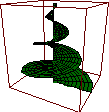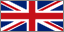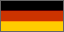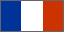About Euler...
News
Screenshots
Documentation
Reference
Examples
Download
Message board
Links
Contact

# Welcome to Euler for GTK+ ...

This is the GTK+ based version of EULER for Unix / Linux systems. It has been ported to GTK+ by Eric Boucharť on the base of Dr Rene Grothmann's X11 version. Euler was started by Dr Grothmann. He also maintains a version for Windows systems.

EULER is a program for quickly and interactively computing with real and complex numbers and matrices, or with intervals, in the style of MatLab, Octave,... It can draw and animate your functions in two and three dimensions.

Euler features :

• real, complex and interval scalars and matrices,
• a programming language, with local variables, default values for parameters, variable parameter number, passing of functions,
• two and three dimensional graphs,
• marker plots,
• density and contour plots,
• animations,
• numerical integration and differentiation,
• statistical functions and tests,
• differential equations,
• interval methods with guaranteed inclusions,
• function minimizers (Brent, Nelder-Mean),
• Simplex algorithm,
• interpolation and approximation,
• finding roots of polynomials,
• Fast Fourier transform (FFT),
• an exact scalar product using a long accumulator,
• Postscript graphics export

and lots more. To meet the Windows version, it still needs some work around the following items :

• calling of functions in external DLLs,
• sound playing.

So, what is it for ?

Suppose you have a non-trivial function and you want to discuss it. You could use one of the plot commands of EULER to get a sketch of the function. Then there are tools to determine zeros or local extrema of the function. You could compute its integral. You could even produce plots of this function with a varying parameter (as a set of plots or as a three dimensional plot).

As another example, suppose you have data contained in a file. EULER can read these data form the file and produce plots of these data, fit polynomials to it, do further computations etc.

In a last example, we assume you have a numerical algorithm to test. You could provide a prototype of this algorithm in the EULER programming language. This is usually done much quicker than using a classical programming language. Furthermore it is interactive and you can use graphics to verify the algorithm.

EULER is an ideal tool for the tasks such as:

• Inspecting and discussing functions of one real or complex variable.
• Viewing surfaces in parameter representation.
• Linear algebra and eigenvalue computation.
• Testing numerical algorithms.
• Solving differential equations numerically.
• Computing polynomials.
• Studying interval arithmetic.
• Examining and generating sound files.

The drawback is that you will have to learn a language. It is not a difficult language. However, there is some learning involved.